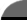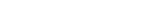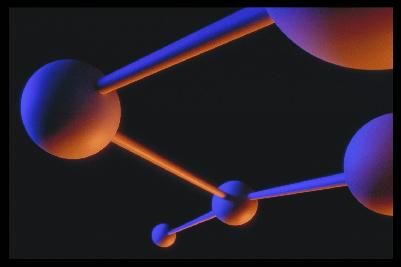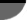Facts By Category:» Physics  » Astronomy  » Chemistry  » Biology  » Mathematics  » Geology  » Engineering  » Medicine  » Science  ScienceIQ Team:»Writers & EditorsxUmp.com
Science Supplies,

Physics & Astronomy
Online
Kinetic Theory of GasesAir is a gas, and gases can be studied by considering the small scale action of individual molecules or by considering the large scale action of the gas as a whole. We can directly measure, or sense, the action of the gas. But to study the action of the molecules, we must use a theoretical model. The model, called the kinetic theory of gases, assumes that the molecules are very small relative to the distance between molecules. The molecules are in constant, random motion and frequently collide with each other and with the walls of any container.

The individual molecules possess the standard physical properties of mass, momentum, and energy. The density of a gas is simply the sum of the mass of the molecules divided by the volume which the gas occupies. The pressure of a gas is a measure of the linear momentum of the molecules. As the gas molecules collide with the walls of a container, the molecules impart momentum to the walls, producing a force that can be measured. The force divided by the area is defined to be the pressure. The temperature of a gas is a measure of the mean kinetic energy of the gas. The molecules are in constant random motion, and there is an energy (mass x square of the velocity) associated with that motion. The higher the temperature, the greater the motion.

In a solid, the location of the molecules relative to each other remains almost constant. But in a gas, the molecules can move around and interact with each other and with their surroundings in different ways. As mentioned above, there is always a random component of molecular motion. The entire fluid can be made to move as well in an ordered motion (flow). The ordered motion is superimposed, or added to, the normal random motion of the molecules. At the molecular level, there is no distinction between the random component and the ordered component. In a pitot tube, we measure pressure produced by the random component as the static pressure, and the pressure produced by the random plus the ordered component as the total pressure.

428

Fact Credit:
NASA Glenn Research Center
Glenn Research Center Web SiteKinetic Theory and Fluid Dynamicsby Yoshio Sone
 Home | Privacy Policy | Cookie PolicyCopyright © 2002-2020 ScienceIQ.com - All Rights Reserved